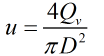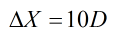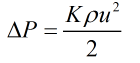# C099 – ISO 3171:1999 Annex A – Estimating Water-in-Oil Dispersion

## Description

This calculation can be used for various piping configurations to indicate whether the dispersion of water in oil is likely to be adequate for sampling to take place.

BS EN ISO 3171:1999 – Petroleum liquids – Automatic pipeline sampling

Kelton calculation reference C099

FLOCALC calculation reference F095

## Options

### Calculate

• Required Energy
• Water concentration ratio (C1/C2)

This option is used to select whether to calculate the water concentration ratio or the required energy for dispersion.

### Method

• Method A
• Method B

This option is used to select how the energy available will be calculated.

### Piping Element (Method A)

• Contraction
• Enlargement
• Orifice
• Circular mitre bends
• Swing check valve
• Angle valve
• Globe valve
• Gate valve

This option is used to select the piping element under consideration when Method A is selected.

### Piping Element (Method B)

• Contraction
• Enlargement
• Bends
• Centrifugal pump
• Throttling valve
• Flow nozzle
• Straight pipe

This option is used to select the piping element under consideration when Method B is selected.

### Pressure Drop

• User entered
• Calculated

If Method A is selected then the pressure drop across the piping element can be user entered or calculated.

### Dissipation Distance

• User entered
• Calculated

If Method A is selected then this option is used to specify whether the dissipation distance is user entered or calculated.

### No. of Bends

• 1
• 2
• 3
• 4
• 5

If bends is selected from the Method B list then this option is used to specify the number of bends in the pipe.

## Calculation

### Flow Velocity

The velocity of the flow through the pipe is calculated by:Where Qv = Gross volumetric flow rate D = Pipe diameter

### Dissipation Distance

In most cases the distance in which the energy has dissipated is not known. Wherever possible the value used should be supported by experimental data. If ΔX is not known it is calculated using the approximation:### Pressure Drop

The pressure drop across the piping element is calculated by:Where ρ = Liquid density K = Resistance coefficient of piping element

### Energy Available

#### Method A

If Method A is selected then the energy available for dispersion is calculated by:#### Method B

##### For Contractions, enlargements and Bends

For these elements the energy available for dispersion is calculated by:Where β = Characteristic parameter of mixing element E0 = Energy dispersion for a straight pipe
##### Centrifugal Pump

If the element selected from the method B list is a centrifugal pump then energy available for dissipation is calculated by:##### Throttle Valve

If the element selected from the method B list is a throttling valve then energy available for dissipation is calculated by:##### Flow Nozzle

If the element selected from the method B list is a nozzle then energy available for dissipation is calculated by:Where φ = Nozzle diameter ue = Velocity at nozzle exit

### Turbulence characteristic

The turbulence characteristic is calculated from the relationship:Where ν = Kinematic viscosity u = Flow velocity

### Settling Rate

The settling rate found via the relationship:Where ρd = Water density

### Energy Required

The energy required for dissipation is calculated from the relationship:If the energy required is less than the energy available then it follows that mixing has not been sufficient for adequate sampling to take place.

Back